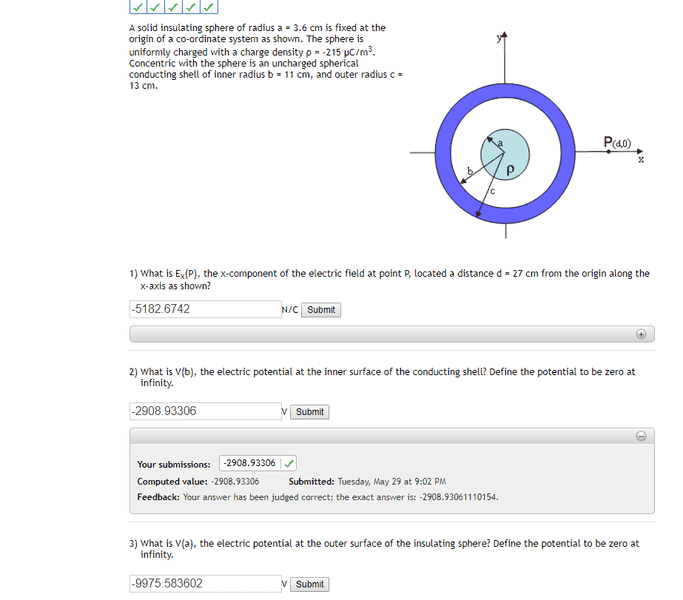# Electric Potential of a Sphere at Different Locations

jakeginobi

## Homework Statement

A solid insulating sphere of radius a = 3.6 cm is fixed at the origin of a co-ordinate system as shown. The sphere is uniformly charged with a charge density ρ = -215 μC/m3. Concentric with the sphere is an uncharged spherical conducting shell of inner radius b = 11 cm, and outer radius c = 13 cm.

I computed the right values for Question 2) and 3), but for Question 2
I don't understand why I have to integrate over infinity to c = 13 cm, why can't I integrate from the origin of the insulating sphere to b since Question 2 is asking for inner surface of conducting shell?

Question 3)
I don't understand why I have to integrate E potential from infinity to C then add integration from B to A.
Couldn't I just integrate from the origin to a to get the outer surface? Is it because the potential is zero at infinity which is the starting point?

## Homework Equations

Integral of (electric field dl)

## The Attempt at a Solution

Question 2) k*(-215*10^-6)(4/3pi(0.0036)^3) * 1/c
Question 3) k*(-215*10^-6)(4/3pi(0.0036)^3) * 1/c + k*(-215*10^-6)(4/3pi(0.0036)^3) * (1/a - 1/b)#### Attachments

•